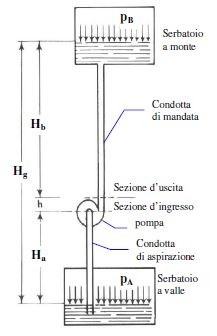Pumps play a fundamental role in transferring fluids.

There are two main factors in determining the size of the pump:

• Flow rate, the quantity of fluid (or volume) that goes through the pump in a specific unit of time.
• Hydraulic head of a pump,  the actual energy that the pump can deliver to the fluid

By examining a generic system like the one shown in the adjacent figure, there are two fundamental measurements:

• Ha head in the suction duct
• Hb head in the delivery duct
• h difference in height between the inlet and outlet of the pump
• pa pressure on the downstream tank
• pb pressure on the upstream tank

## CALCULATING THE HEAD OF A PUMP: BERNOULLI’S PRINCIPLE

What is the head of a pump? As mentioned earlier, the head corresponds to the actual energy that the pump delivers to the fluid. The Bernoulli equation is applied between the pump’s inlet and outlet sections: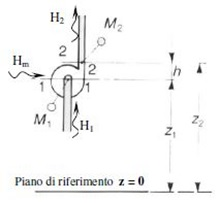H1 + Hm = H2       H1 = inlet energy
Hm = energy delivered by the pump
H2 = outlet energy

Replacing with Bernoulli’s equation: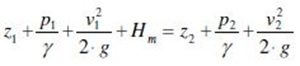The term that we normally want to find is Hm.
However, during the design stage, P1 and P2 are never known (as there is no physical element yet and therefore it is not possible to effectively measure the pump’s inlet and outlet pressure).

Therefore two new terms are defined, which are known, and which allow us to overcome the problem and determine the size of the pump:
P1 = Pa – γ * Ha
P2 = Pb + γ * Hb

Considering, moreover, that:
Hg = h + Ha + Hb

Which gives us a relation defined as follows: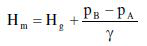## CALCULATING THE HEAD LOSS FOR A PUMP

In a real situation we must also consider the head loss due to the fluid flowing through the ducts and in the various construction parts, in suction and delivery (valves, curves, fittings, etc.).

The equation is defined as follows: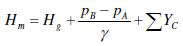Now we will examine a more complex issue, that is the calculation of the last term of the aforementioned equation, which represents the total of the losses distributed along the pipes (suction and delivery) and the concentrated losses (valves, curves, etc.).

As the fluid flows inside a pipe it loses energy (deducible in terms of pressure) due to the friction between the fluid and the walls of the pipe.
These losses depend on the characteristics of the pipe and a re proportional to its length.

In order to calculate them we must define a friction factor, which depends on the speed of the fluid.
There are two different cases: laminar flow and turbulent flow.

### 1. LAMINAR FLOW

The flow of the fluid is laminar, or Reynolds < 2000.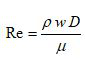Ρ = fluid density
W = fluid speed
D = fluid diameter
µ = fluid viscosity

where the speed can be deduced by knowing the flow rate necessary for the size and therefore from the following formula:
w = (4*Q)/(π*D2)

The friction factor in this type of flow is defined as follows:
Fa = 64/Re
Therefore to calculate the continuous losses expressed in metres, in laminar flow, we will proceed as follows:
Yc = (Fa/ γ) *(L/D) * w2/2

### 2. TURBULENT FLOW

The flow of the fluid is turbulent, or Reynolds > 4000, (most common case).
Taking into account the formulas used to calculate the speed and the Re number, the resulting friction factor will be:

Fa = 0.07 * Re-0.13 * D-0.14

And the head losses in the turbulent flow will be:

Yc = (Fa/ γ) *(L/D) * w2/2

Along the pipes of a system there are numerous construction and management elements such as valves, fittings, narrowing, etc., that contribute to head loss.
There are different methods for calculating these head losses.
The simplest and easiest to understand is by using the following diagram.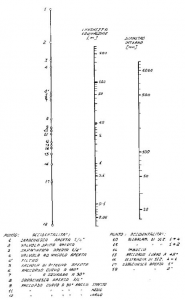• The axis on the right represents the internal diameter of the pipe expressed in mm.
• The axis on the left represents the different obstacles along the pipe.
• The axis in the middle is the corresponding head loss expressed in metres, relative to the element in question (e.g. valve), along a pipe with an internal D of 50 mm.

At this point we can easily calculate the head losses of the system, and therefore choose the correct size of the pump to achieve the desired flow rate at the resulting equivalent head.